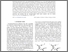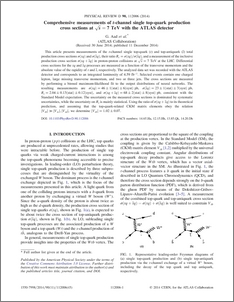# Comprehensive measurements of t-channel single top-quark production cross sections at s√=7 TeV with the ATLAS detector

, The ATLAS collaboration (2014) Comprehensive measurements of t-channel single top-quark production cross sections at s√=7  TeV with the ATLAS detector. Physical Review D, 90 (11). ISSN 1550-7998Preview
PDF (PhysRevD.90.112006)
PhysRevD.90.112006.pdf - Published Version

## Abstract

This article presents measurements of the t-channel single top-quark (t) and top-antiquark (t¯) total production cross sections σ(tq) and σ(t¯q), their ratio Rt=σ(tq)/σ(t¯q), and a measurement of the inclusive production cross section σ(tq+t¯q) in proton-proton collisions at s√=7  TeV at the LHC. Differential cross sections for the tq and t¯q processes are measured as a function of the transverse momentum and the absolute value of the rapidity of t and t¯, respectively. The analyzed data set was recorded with the ATLAS detector and corresponds to an integrated luminosity of 4.59  fb−1. Selected events contain one charged lepton, large missing transverse momentum, and two or three jets. The cross sections are measured by performing a binned maximum-likelihood fit to the output distributions of neural networks. The resulting measurements are σ(tq)=46±1(stat)±6(syst)  pb, σ(t¯q)=23±1(stat)±3(syst)  pb, Rt=2.04±0.13(stat)±0.12(syst), and σ(tq+t¯q)=68±2(stat)±8(syst)  pb, consistent with the Standard Model expectation. The uncertainty on the measured cross sections is dominated by systematic uncertainties, while the uncertainty on Rt is mainly statistical. Using the ratio of σ(tq+t¯q) to its theoretical prediction, and assuming that the top-quark-related CKM matrix elements obey the relation |Vtb|≫|Vts|,|Vtd|, we determine |Vtb|=1.02±0.07.

Item Type:
Journal Article
Journal or Publication Title:
Physical Review D